Function Repository Resource:

# ChamferedPolyhedron

Chamfer a given polyhedron

Contributed by: Jan Mangaldan
 ResourceFunction["ChamferedPolyhedron"][poly] gives the chamfered polyhedron of poly by chamfering all edges. ResourceFunction["ChamferedPolyhedron"][poly,l] chamfers the polyhedron poly by a length ratio l at its edges.

## Details

Chamfering, or edge truncation, is done by truncating each edge of a polyhedron with the plane perpendicular to the plane bisecting the dihedral angle between two faces, effectively replacing each original edge with a hexagonal face.

## Examples

### Basic Examples (1)

Chamfer a dodecahedron:

 In:=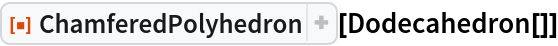Out=In:=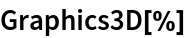Out=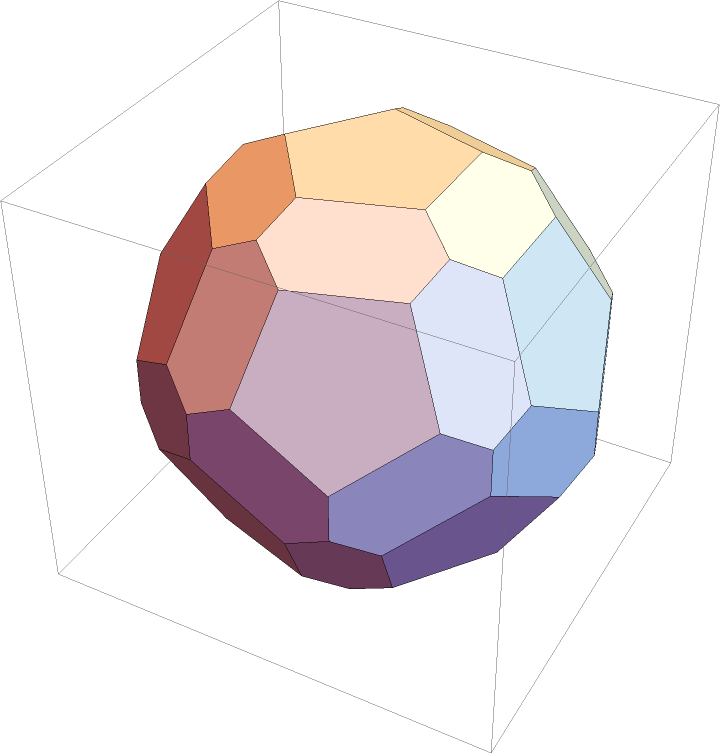### Scope (2)

ChamferedPolyhedron works on polyhedra:

 In:=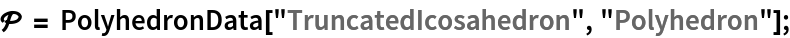In:=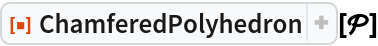Out=In:=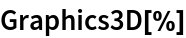Out=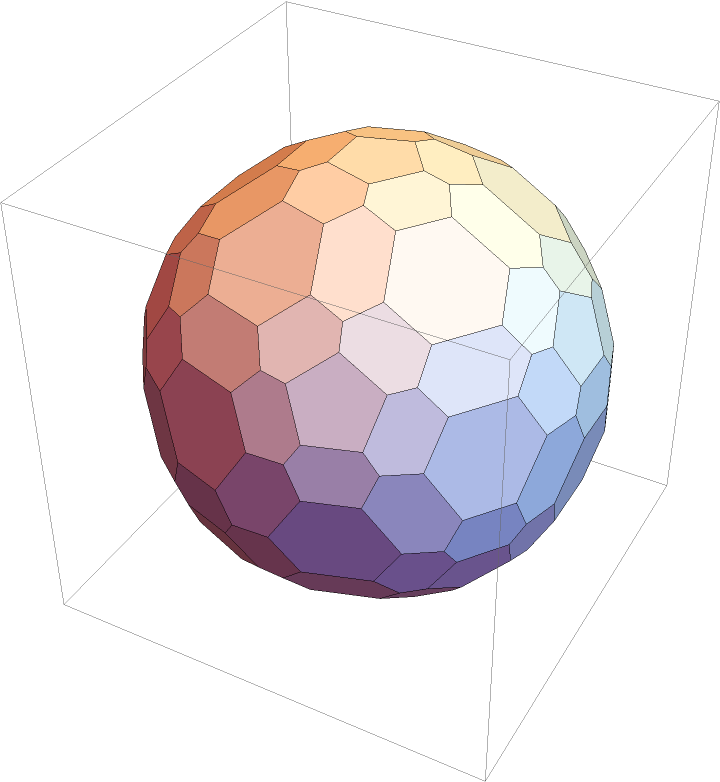Chamfer the polyhedron by different length ratios:

 In:=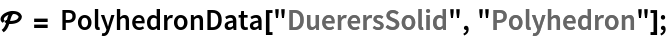In:=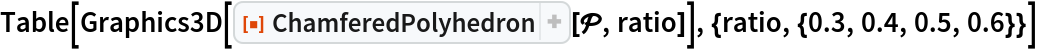Out=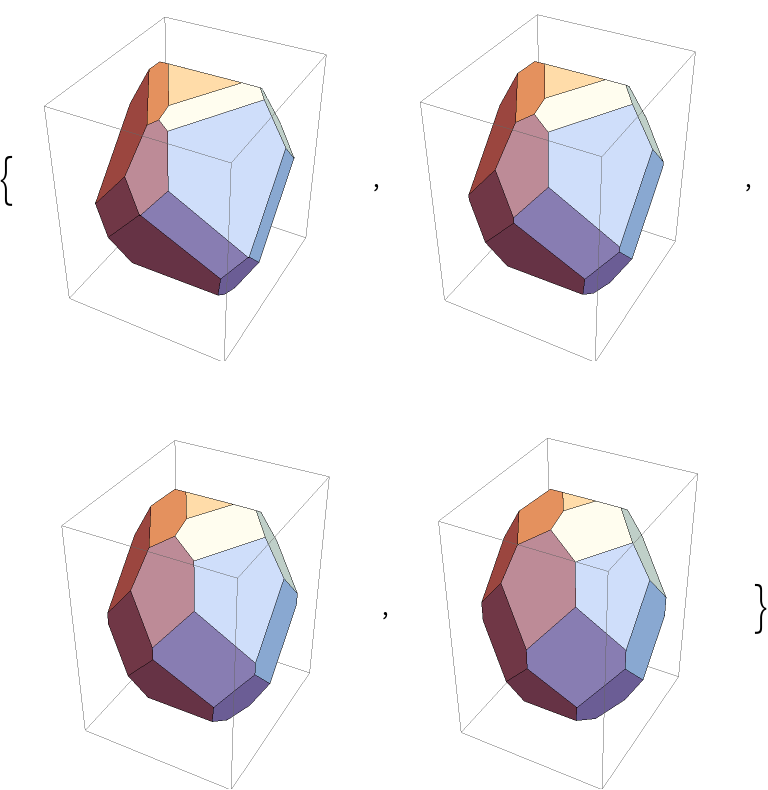### Properties and Relations (1)

ChamferedPolyhedron transforms edges, while BeveledPolyhedron transforms edges and vertices:

 In:=Out=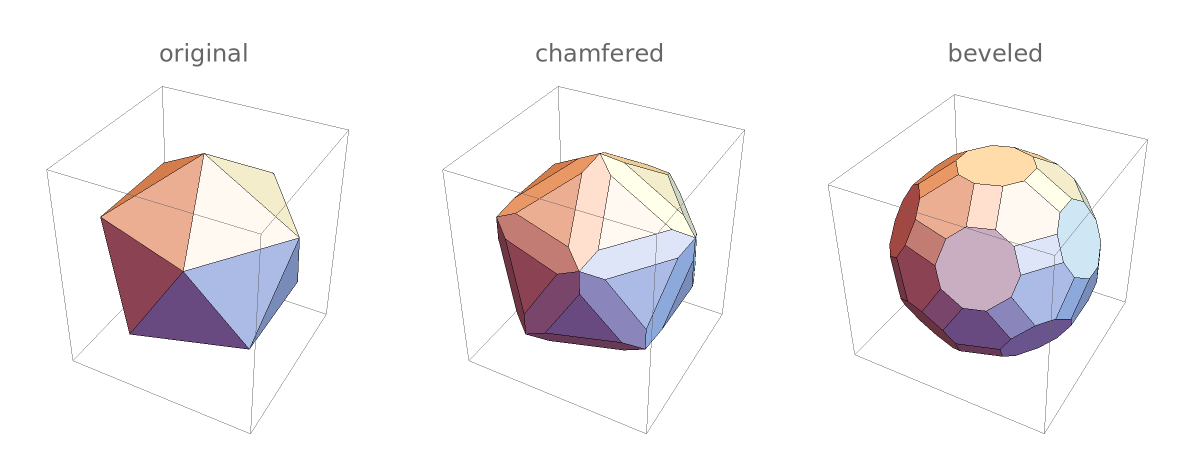### Possible Issues (1)

ChamferedPolyhedron only supports simple polyhedra:

 In:=Out=In:=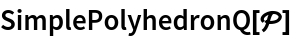Out=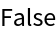In:=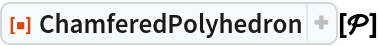Out=### Neat Examples (2)

Equilateral chamfered polyhedra based on Platonic solids:

 In:=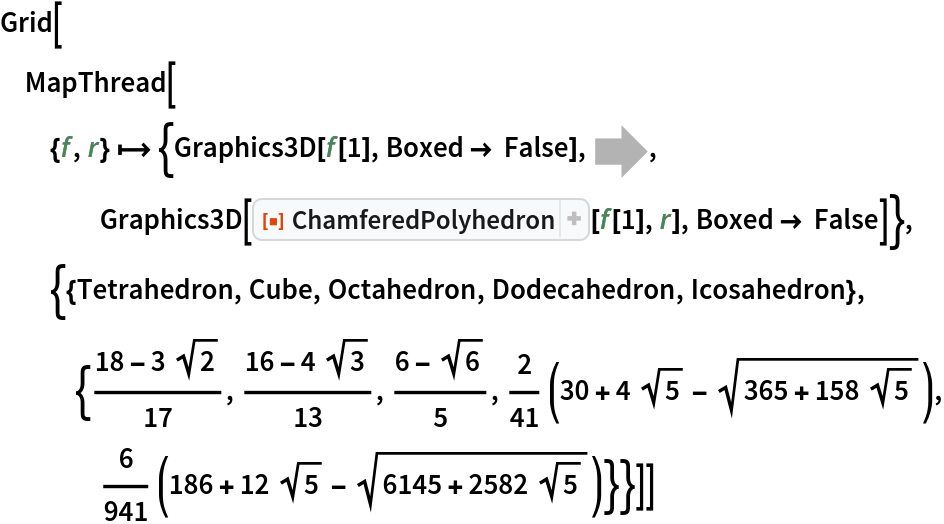Out=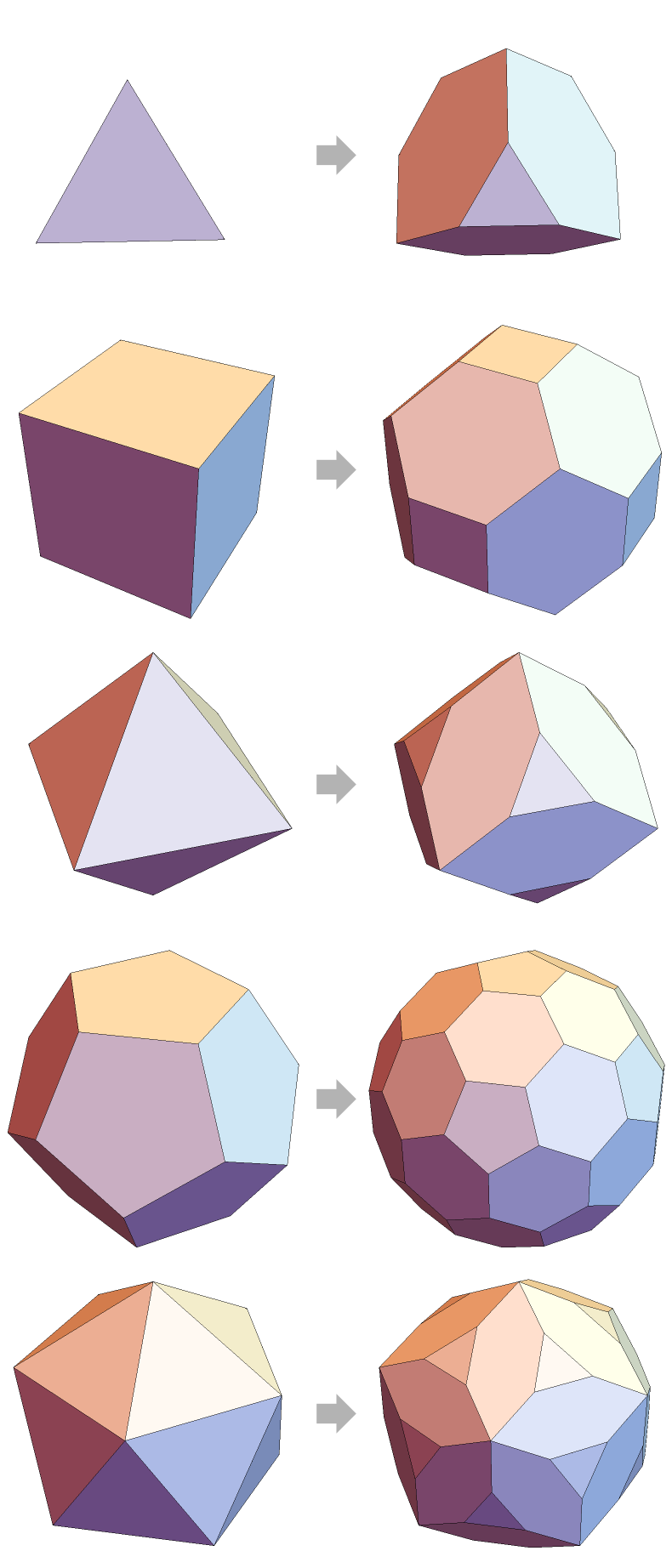Show the relationship between a polyhedron and its chamfered version:

 In:=Out=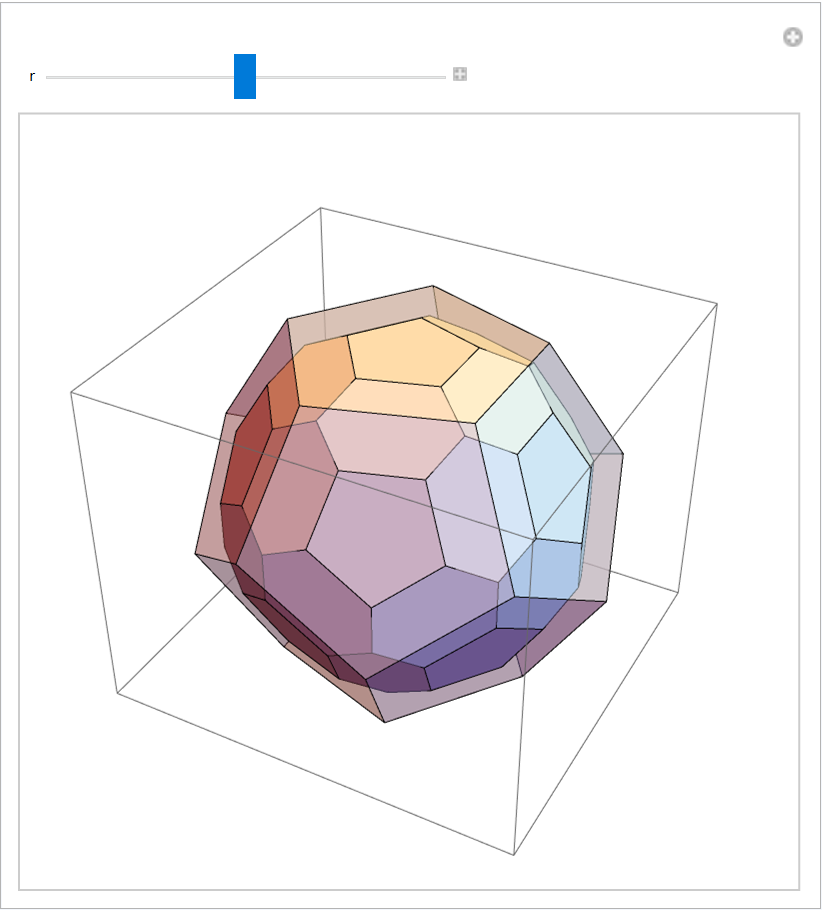## Version History

• 1.0.0 – 21 September 2021